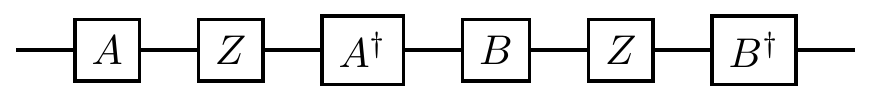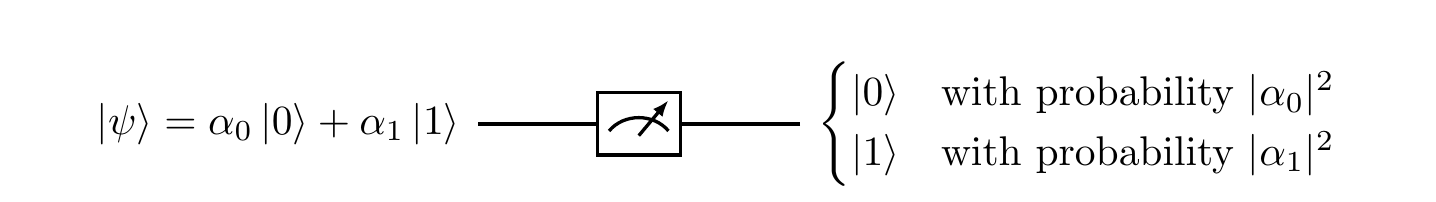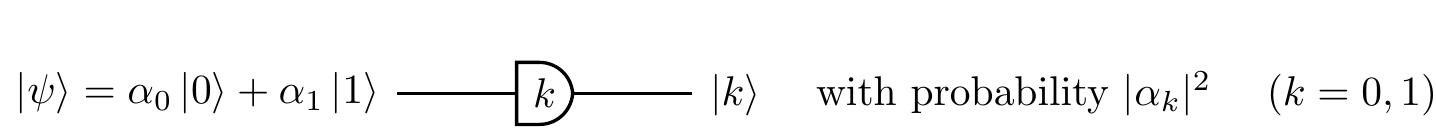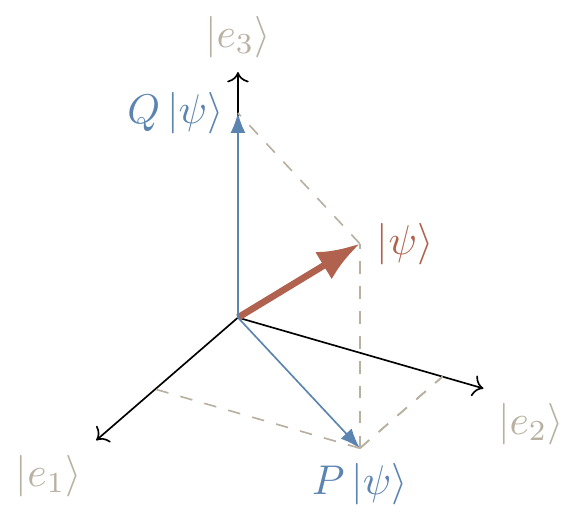## 5.6 Controlled-NOT

How do entangled states arise in real physical situations? The short answer is that entanglement is the result of interactions. It is easy to see that tensor product operations U_1\otimes\ldots\otimes U_n map product states to product states:and so any collection of separable qubits remains separable. As soon as qubits start interacting with one another, however, they become entangled, and things start to get really interesting. We will describe interactions that cannot be written as tensor products of unitary operations on individual qubits.

The most popular two-qubit entangling gate is the controlled-\texttt{NOT} (or \texttt{c-NOT}), also known as the controlled-X gate.93 The gate acts on two qubits: it flips the second qubit (referred to as the target) if the first qubit (referred to as the control) is |1\rangle, and does nothing if the control qubit is |0\rangle. In the standard basis \{|00\rangle,|01\rangle,|10\rangle,|11\rangle\}, it is represented by the following unitary matrix:

controlled-\texttt{NOT}
\left[\begin{array}{c|c}\begin{matrix}1&0\\0&1\end{matrix}&\begin{matrix}0&0\\0&0\end{matrix}\\\hline\begin{matrix}0&0\\0&0\end{matrix}&\begin{matrix}0&1\\1&0\end{matrix}\end{array}\right]

We represent the \texttt{c-NOT} gate in circuit notation as shown in Figure 5.1.Figure 5.1: Where x,y\in\{0,1\}, and \oplus denotes \texttt{XOR}, or addition modulo 2.

Note that this gate does not admit any tensor product decomposition, but can be written as a sum of tensor products:94 \texttt{c-NOT} = |0\rangle\langle 0|\otimes\mathbf{1}+ |1\rangle\langle 1|\otimes X (where X is the Pauli bit-flip operation).

The \texttt{c-NOT} gate lets us do many interesting things, and can act in a rather deceptive way. Let us now study some of these things.

### 5.6.1 The Bell states, and the Bell measurement

We start with the generation of entanglement. Here is a simple circuit that demonstrates the entangling power of \texttt{c-NOT}:95

(Generating entanglement).In this circuit, the separable input |0\rangle|0\rangle evolves as \begin{aligned} |0\rangle|0\rangle \overset{H}{\longmapsto}& \frac{1}{\sqrt{2}} (|0\rangle + |1\rangle) |0\rangle \\=& \frac{1}{\sqrt{2}}|0\rangle|0\rangle + \frac{1}{\sqrt{2}}|1\rangle|0\rangle \\\overset{\texttt{c-NOT}}{\longmapsto}& \frac{1}{\sqrt{2}}|0\rangle|0\rangle + \frac{1}{\sqrt{2}}|1\rangle|1\rangle \end{aligned} resulting in the entangled output \frac{1}{\sqrt{2}}(|00\rangle+|11\rangle). In fact, this circuit implements the unitary operation which maps the standard computational basis into the four entangled states, known as the Bell states.

The Bell states |\psi_{ij}\rangle are those generated by the above circuit: \begin{aligned} |00\rangle &\longmapsto |\psi_{00}\rangle \coloneqq \frac{1}{\sqrt{2}}(|00\rangle+|11\rangle) \\|01\rangle &\longmapsto |\psi_{01}\rangle \coloneqq \frac{1}{\sqrt{2}}(|01\rangle+|10\rangle) \\|10\rangle &\longmapsto |\psi_{10}\rangle \coloneqq \frac{1}{\sqrt{2}}(|00\rangle-|11\rangle) \\|11\rangle &\longmapsto |\psi_{11}\rangle \coloneqq \frac{1}{\sqrt{2}}(|01\rangle-|10\rangle) \end{aligned} The more standard notation for these states, however, is the following: \begin{aligned} \Phi^+ &\coloneqq |\psi_{00}\rangle \\\Psi^+ &\coloneqq |\psi_{01}\rangle \\\Phi^- &\coloneqq |\psi_{10}\rangle \\\Psi^-&\coloneqq |\psi_{11}\rangle \end{aligned} (and this is the notation that we will use from now on).

The Bell states form an orthonormal basis in the Hilbert space \mathcal{H}_1\otimes\mathcal{H}_2 of two qubits. We can perform measurements in the Bell basis: the easiest way to do it in practice is to “rotate” the Bell basis to the standard basis, and then perform the measurement in the standard basis.96 Indeed, if we reverse the circuit (running it from right to left), then we get a circuit which maps the Bell state |\psi_{ij}\rangle to the corresponding state |ij\rangle in the standard basis. This unitary mapping allows us to “implement” the projections on Bell states by applying the reversed circuit followed by the usual qubit-by-qubit measurement in the standard basis.

### 5.6.2 Quantum teleportation

A wonderful fact, that sounds more like science fiction than actual science, is the following: an unknown quantum state can be teleported from one location to another. Consider the following circuit, which is built from a Bell state generator followed by an “offset” inverse Bell state generator:97

(Quantum teleportation).The first input qubit (counting from the top) is in some arbitrary state. After the action of the part of the circuit in the first dashed box (counting from the left), the state of the three qubits reads98 \big( \alpha|0\rangle+\beta|1\rangle \big) \big( |00\rangle+|11\rangle \big). By regrouping the terms, but keeping the qubits in the same order, this state can be written as the sum \begin{aligned} &(|00\rangle + |11\rangle) \otimes (\alpha|0\rangle + \beta|1\rangle) \\+ &(|01\rangle + |10\rangle) \otimes (\alpha|1\rangle + \beta|0\rangle) \\+ &(|00\rangle - |11\rangle) \otimes (\alpha|0\rangle - \beta|1\rangle) \\+ &(|01\rangle - |10\rangle) \otimes (\alpha|1\rangle - \beta|0\rangle). \end{aligned} Then the part of the circuit in the second dashed box maps the four Bell states of the first two qubits to the corresponding states from the computational basis: \begin{aligned} &|00\rangle \otimes (\alpha|0\rangle + \beta|1\rangle) \\+ &|01\rangle \otimes (\alpha|1\rangle + \beta|0\rangle) \\+ &|10\rangle \otimes (\alpha|0\rangle - \beta|1\rangle) \\+ &|11\rangle \otimes (\alpha|1\rangle - \beta|0\rangle). \end{aligned} Upon performing the standard measurement and learning the values of x and y, we choose one of the four following transformations depending on these values: \begin{array}{ll} 00 \mapsto \mathbf{1} &\quad 01 \mapsto X \\[1em] 10 \mapsto Z &\quad 11 \mapsto ZX \end{array} \tag{$\circledast$} (e.g. if x=0 and y=1, then we choose X). We then apply this transformation to the third qubit, which restores the original state of the first qubit.

If you understand how this circuit works99, then you are ready for quantum teleportation. Here is a dramatic version.

Suppose that three qubits, which all look very similar, are initially in the possession of an absent-minded Oxford student, Alice. The first qubit is in a precious quantum state and this state is needed urgently for an experiment in Cambridge. The other two qubits are entangled, in the \Phi^+=|\psi_{00}\rangle state. Alice’s colleague, Bob, pops in to collect the qubit. Once he is gone, Alice realises that, by mistake, she gave him not the first but the third qubit: the one which is entangled with the second qubit.The situation seems to be hopeless — Alice does not know the quantum state of the first qubit, and Bob is now miles away and her communication with him is limited to few bits. However, Alice and Bob are both very clever and they both diligently attended their “Introduction to Quantum Information Science” classes. Can Alice rectify her mistake and save Cambridge science?

Hmm… (pause for thought)

Of course: Alice can teleport the state of the first qubit! She performs the Bell measurement on the first two qubits, which gives her two binary digits, x and y. She then broadcasts x and y to Bob, who chooses the corresponding transformation, as in Equation (\circledast), performs it, and recovers the original state.

This raises a natural “philosophical” question: what do we really mean by teleportation? A key part of this question is understanding what happens to our original qubit when we teleport it. Note that the actual physical electron (or whatever implementation of qubits we are using) does not suddenly move through space — what is teleported is the state of the qubit, but the argument can be made that if two qubits are entirely indistinguishable from one another by any measurements that we can make, then they really are “the same” in every way that matters, and so the qubit which now has the original qubit’s state “is the same as” the original qubit. As it turns out, this process necessarily destroys the original qubit’s state, as we now explain.

The first actual teleportation experiment was successfully achieved in 1997 (arXiv:quant-ph/9710013); in 2012 a record distance was set: an entangled photon pair was used to teleport a state 143 kilometres/88 miles (arXiv:1205.3909); in 2017, successful ground-to-satellite teleportation was achieved (arXiv:). This is not science fiction!

But there is a fundamental question to ask: if the original state is destroyed, then how can we really verify that teleportation has taken place? We can’t compare the purportedly teleported state to the original one! The answer to this involves certain no-go theorems and statistical methods, where we can show that classical physics gives some strict upper bound on a certain fidelity, but which is clearly surpassed by these physical experiments. We will better explain the ideas behind these sorts of arguments later on, in Section 10, when we introduce Bell’s theorem.

### 5.6.3 Thou shalt not clone

Let us now look at something that the controlled-\texttt{NOT} seems to be doing but, in fact, isn’t. It is easy to see that the \texttt{c-NOT} can copy the bit value of the first qubit: |x\rangle|0\rangle \overset{\texttt{c-NOT}}{\longmapsto} |x\rangle|x\rangle \qquad\text{(for $x=0,1$)} so one might suppose that this gate could also be used to copy superpositions, such as |\psi\rangle = \alpha|0\rangle+\beta|1\rangle, so that |\psi\rangle|0\rangle \overset{\texttt{c-NOT}}{\longmapsto} |\psi\rangle|\psi\rangle for any |\psi\rangle.

This is not true!

The unitarity of the \texttt{c-NOT} means that it turns superpositions in the control qubit into entanglement of the control and the target: if the control qubit is in the a superposition state |\psi\rangle = \alpha|0\rangle+\beta|1\rangle (with \alpha,\beta\neq0), and the target is in |0\rangle, then the \texttt{c-NOT} gate generates the entangled state \big( \alpha|0\rangle+\beta|1\rangle \big) |0\rangle \overset{\texttt{c-NOT}}{\longmapsto} \alpha|00\rangle + \beta|11\rangle. In fact, it is impossible to clone an unknown quantum state, and we can prove this!

To prove this via contradiction, let us assume that we could build a universal quantum cloner, and then take any two normalised states |\psi\rangle and |\phi\rangle that are non-identical (i.e. |\langle\psi|\phi\rangle|\neq1) and non-orthogonal (i.e. \langle\psi|\phi\rangle\neq0). If we then run our hypothetical cloning machine we get \begin{aligned} |\psi\rangle|0\rangle|W\rangle &\mapsto |\psi\rangle|\psi\rangle|W'\rangle \\|\phi\rangle|0\rangle|W\rangle &\mapsto |\phi\rangle|\phi\rangle|W''\rangle \end{aligned} where the third system, initially in state |W\rangle, represents everything else (say, the internal state of the cloning machine). For this transformation to be unitary, it must preserve the inner product, and so we require that \langle\psi|\phi\rangle = \langle\psi|\phi\rangle^2 \langle W'|W''\rangle which can only be satisfied if |\langle\psi|\phi\rangle| is equal to 1 or 0, but this contradicts our assumptions!

Thus, states of qubits, unlike states of classical bits, cannot be faithfully cloned. Note that, in quantum teleportation, the original state must therefore be destroyed, since otherwise we would be producing a clone of an unknown quantum state. The no-cloning property of quantum states leads to interesting applications, of which quantum cryptography is one.

Universal quantum cloners are impossible.

1. Here, X\equiv\sigma_x refers to the Pauli operator that implements the bit-flip.↩︎

2. Make sure that you understand how the Dirac notation is used here. More generally, think why |0\rangle\langle 0|\otimes A + |1\rangle\langle 1|\otimes B means “if the first qubit is in state |0\rangle then apply A to the second one, and if the first qubit is in state |1\rangle then apply B to the second one”. What happens if the first qubit is in a superposition of |0\rangle and |1\rangle?↩︎

3. John Stewart Bell (1928–1990) was a Northern Irish physicist.↩︎

4. For any state |\psi\rangle of two qubits, the amplitude \langle\psi_{ij}|\psi\rangle can be written as \langle ij|U^\dagger|\psi\rangle, where U^\dagger is such that |\psi_{ij}\rangle = U|ij\rangle.↩︎

5. Divide et impera, or “divide and conquer”: a good approach to solving problems in mathematics (and in life). Start with the smaller circuits in the dashed boxes, which we have just seen introduced above.↩︎

6. We don’t worry about writing the normalisation factors.↩︎

7. You can play around with this on the Quantum Flytrap Virtual Lab.↩︎